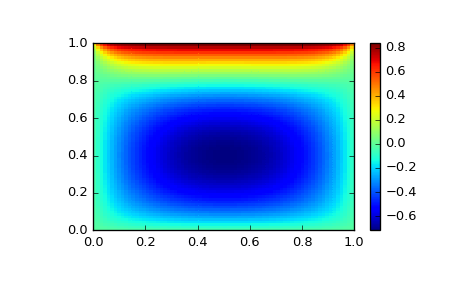# Nonlinear solvers¶

This is a collection of general-purpose nonlinear multidimensional solvers. These solvers find x for which F(x) = 0. Both x and F can be multidimensional.

## Routines¶

Large-scale nonlinear solvers:

 newton_krylov(F, xin[, iter, rdiff, method, ...]) Find a root of a function, using Krylov approximation for inverse Jacobian. anderson(F, xin[, iter, alpha, w0, M, ...]) Find a root of a function, using (extended) Anderson mixing.

General nonlinear solvers:

 broyden1(F, xin[, iter, alpha, ...]) Find a root of a function, using Broyden’s first Jacobian approximation. broyden2(F, xin[, iter, alpha, ...]) Find a root of a function, using Broyden’s second Jacobian approximation.

Simple iterations:

 excitingmixing(F, xin[, iter, alpha, ...]) Find a root of a function, using a tuned diagonal Jacobian approximation. linearmixing(F, xin[, iter, alpha, verbose, ...]) Find a root of a function, using a scalar Jacobian approximation. diagbroyden(F, xin[, iter, alpha, verbose, ...]) Find a root of a function, using diagonal Broyden Jacobian approximation.

## Examples¶

Small problem

>>> def F(x):
...    return np.cos(x) + x[::-1] - [1, 2, 3, 4]
>>> import scipy.optimize
>>> x = scipy.optimize.broyden1(F, [1,1,1,1], f_tol=1e-14)
>>> x
array([ 4.04674914,  3.91158389,  2.71791677,  1.61756251])
>>> np.cos(x) + x[::-1]
array([ 1.,  2.,  3.,  4.])


Large problem

Suppose that we needed to solve the following integrodifferential equation on the square $$[0,1]\times[0,1]$$:

$\nabla^2 P = 10 \left(\int_0^1\int_0^1\cosh(P)\,dx\,dy\right)^2$

with $$P(x,1) = 1$$ and $$P=0$$ elsewhere on the boundary of the square.

The solution can be found using the newton_krylov solver:

import numpy as np
from scipy.optimize import newton_krylov
from numpy import cosh, zeros_like, mgrid, zeros

# parameters
nx, ny = 75, 75
hx, hy = 1./(nx-1), 1./(ny-1)

P_left, P_right = 0, 0
P_top, P_bottom = 1, 0

def residual(P):
d2x = zeros_like(P)
d2y = zeros_like(P)

d2x[1:-1] = (P[2:]   - 2*P[1:-1] + P[:-2]) / hx/hx
d2x    = (P    - 2*P    + P_left)/hx/hx
d2x[-1]   = (P_right - 2*P[-1]   + P[-2])/hx/hx

d2y[:,1:-1] = (P[:,2:] - 2*P[:,1:-1] + P[:,:-2])/hy/hy
d2y[:,0]    = (P[:,1]  - 2*P[:,0]    + P_bottom)/hy/hy
d2y[:,-1]   = (P_top   - 2*P[:,-1]   + P[:,-2])/hy/hy

return d2x + d2y - 10*cosh(P).mean()**2

# solve
guess = zeros((nx, ny), float)
sol = newton_krylov(residual, guess, method='lgmres', verbose=1)
print('Residual: %g' % abs(residual(sol)).max())

# visualize
import matplotlib.pyplot as plt
x, y = mgrid[0:1:(nx*1j), 0:1:(ny*1j)]
plt.pcolor(x, y, sol)
plt.colorbar()
plt.show()